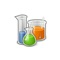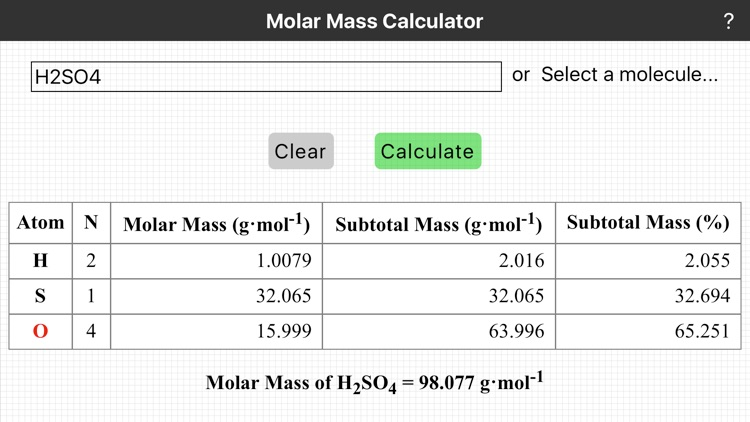You are using an outdated browser. Please upgrade your browser to improve your experience.## In chemistry the numbers of atoms, molecules, or formula units, amount of substance, in a sample is reported in the SI unit “mole” (abbreviated mol)# Chemistry - Molar Mass

by Thundercloud Consulting, LLC### What is it about?

In chemistry the numbers of atoms, molecules, or formula units, amount of substance, in a sample is reported in the SI unit “mole” (abbreviated mol). By definition 1 mole of something contains the same number of objects as there are atoms in exactly 12 g of carbon-12. This number (i.e., 6.0221 x 10^23) is known as Avogadro's constant.Version
1.2.1
Rating
NA
Size
3Mb
Genre
Education
Last updated
March 5, 2017
Release date
March 7, 2012
More info

### App Store Description

In chemistry the numbers of atoms, molecules, or formula units, amount of substance, in a sample is reported in the SI unit “mole” (abbreviated mol). By definition 1 mole of something contains the same number of objects as there are atoms in exactly 12 g of carbon-12. This number (i.e., 6.0221 x 10^23) is known as Avogadro's constant.

A chemical substance may consist of atoms of a single element (e.g., O2), or of different elements (e.g., CO2). The molar mass of an element is the mass per mole of its atoms. The molar mass of a compound is the mass per mole of its molecules. The molar mass of an ionic compound is mass per mole of its formula units. To calculate the molar mass of a substance we add up the individual molar masses of the constituent atoms.

Note: In nature a sample of an element contains isotopes. Isotopes are atoms with the same number of protons in the nucleus (atomic number), but different masses. The difference in mass between isotopes is the result of different numbers of neutrons. The value of the molar mass of an element used in chemical calculations takes the natural abundance of isotopes into account (i.e., the molar mass is the weighted sum of the isotopic masses found in a typical sample).

To use this calculator enter a chemical formula and tap OK, or alternatively, select a sample molecule from the menu. For example, if you needed the molar mass of sulfuric acid you would enter H2SO4. Parentheses can also be used: the formula for pentane could be entered as CH3(CH2)3CH3 or CH3CH2CH2CH2CH3.

Disclaimer:
AppAdvice does not own this application and only provides images and links contained in the iTunes Search API, to help our users find the best apps to download. If you are the developer of this app and would like your information removed, please send a request to [email protected] and your information will be removed.